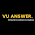# PHY101 Assignment 1 Solution 2021 - VU Answer

A Complete Step by Step PHY101 Assignment 1 Solution Spring 2021. Easy to View PHY101 Assignment Solution 2021. Download Correct PHY101 Assignment Solution PDF File.

Check Here New PHY101 Assignment

PHY101 ASSIGNMENT 1 SOLUTION SPRING 2021

Question 1:

The student of Physics claims that he has calculated a negative potential difference between two points. Is it true or wrong? Either yes or no explain it by giving a suitable example.

Solution:

It is true. If he took the reference potential at a higher point and the other at a lower then the potential difference is negative.

Example.

The reference potential is 5v and the other is 3 V then the potential difference is – 2V.

Question 2:Determine the magnitude as well as direction of the electric field at point A, shown in the above figure. Given the value of k = 8.99 × 1012N/C.

Solution:Question 3:

In the figure shown, the lamp cord is 85cm long and comprises copper wire. Calculate the wire‘s resistance? Use a value of resistivity for Cu as 1.75 × 10-8Ωm.Solution:1. Don't copy-paste the same answer.

2. Make sure you can make some changes to your solution file before submitting copy-paste solution will be marked zero.

3. If you found any mistake then correct yourself and inform me.

4. Before submitting an assignment check your assignment requirement file.

5. If you need some help and question about file and solutions.

1.1.